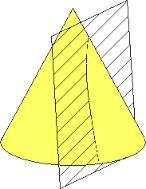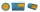# Surface area

The volume of a cone is 1000 cm3 and the content area of the axis cut is 100 cm2. Calculate the surface area of the cone.

Correct result:

S =  711.64 cm2

#### Solution:

$S_1 = 100 \ cm^2 \ \\ V = 1000 \ cm^3 \ \\ S_1 = \dfrac{ 2r h }{2} \ \\ rh = 100 \ \\ h = 100/r \ \\ V = \dfrac13 \pi r^2 h \ \\ V = \dfrac13 \pi r^2 100 /r \ \\ V = \dfrac{ 100 }{3} \pi r \ \\ r = 9.55 \ cm \ \\ h = 100 / r = 10.47 \ cm \ \\ \ \\ s = \sqrt {r^2 + h^2 } = 14.17 \ cm \ \\ \ \\ S = \pi r (r+s) = \pi 9.55 \cdot (9.55+14.17) = 711.64 \ \text{cm}^2 \ \\$We would be pleased if you find an error in the word problem, spelling mistakes, or inaccuracies and send it to us. Thank you!Tips to related online calculators
Tip: Our volume units converter will help you with the conversion of volume units.

## Next similar math problems:

• The stringThey cut 113 cm from the string and divided the rest in a ratio of 5: 6.5: 8: 9.5. The longest part measured 38 cm. Find the original length of the string.
• The cubeThe cube has a surface of 600 cm2, what is its volume?
• Edge cFind the edge c of cuboid if an edge a = 20 mm, b = 30 mm and surface area S = 8000 mm2.
• Cube V2SThe volume of the cube is 27 dm cubic. Calculate the surface of the cube.
• Sphere in coneA sphere is inscribed in the cone (the intersection of their boundaries consists of a circle and one point). The ratio of the surface of the ball and the contents of the base is 4: 3. A plane passing through the axis of a cone cuts the cone in an isoscele
• Edge of prismThe regular quadrilateral prism has a surface of 250 dm2, its shell has a content of 200 dm2. Calculate its leading edge.
• The cylinderThe cylinder has a surface area of 300 square meters, while the height of the cylinder is 12 m. Calculate the volume of this cylinder.How many times does the surface of a sphere decrease if we reduce its radius twice?
• Cube surfce2volumeCalculate the volume of the cube if its surface is 150 cm2.
• The cubeThe cube has a surface area of 216 dm2. Calculate: a) the content of one wall, b) edge length, c) cube volume.The surface of the regular quadrilateral prism is 8800 cm2, the base edge is 20 cm long. Calculate the volume of the prismEight small Christmas balls with a radius of 1 cm have the same volume as one large Christmas ball. What has a bigger surface: eight small balls, or one big ball?Calculate how much cover (without a floor) is used to make a tent that has the shape of a regular square pyramid. The edge of the base is 3 m long and the height of the tent is 2 m.Calculate the surface of a quadrilateral pyramid, which has a rectangular base with dimensions a = 8 cm, b = 6 cm and height H = 10 cm.3750 cm square of wallpaper is needed to glue a cube-shaped box. Can Dad cut out the whole necessary piece of wallpaper as a whole if he has a roll of wallpaper 50 cm wide?The surface of the cylinder, the height of which is equal to the diameter of the base, is 4239 cm square. Calculate the cylinder volume.The regular quadrilateral pyramid has a volume of 24 dm3 and a height of 45 cm. Calculate its surface.